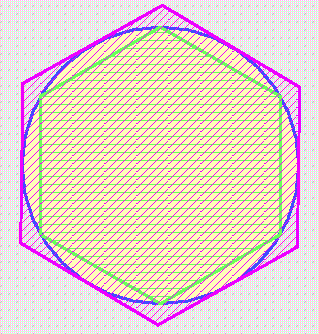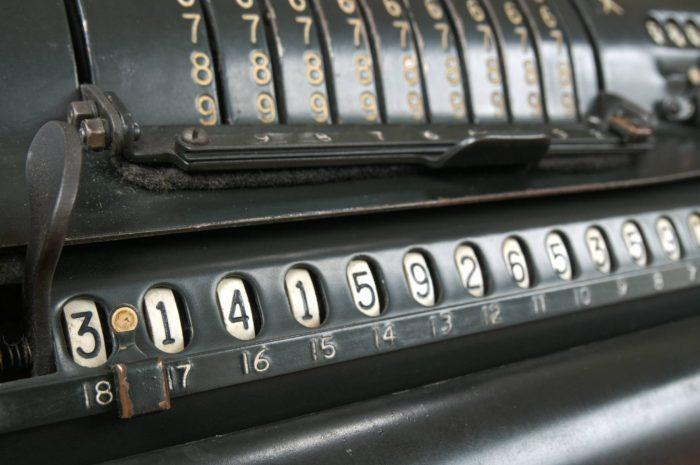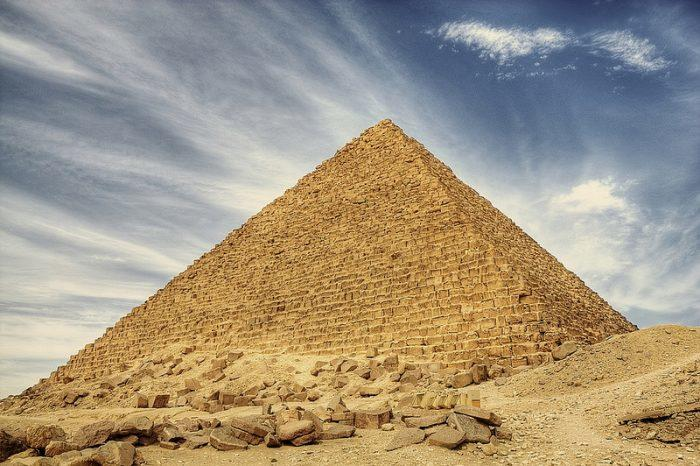Share

The Importance of ‘Pi’ in the World

By Jon, published on 31/03/2018 > > > The Value of Pi in Mathematics

First of all, why Pi?

The mathematical symbol for Pi “π” is a Greek letter, derived from the first letter of the Greek words “περιφέρεια”, meaning periphery, and “περίμετρος”, meaning perimeter.

The earliest known use of the symbol was by Welsh mathematician William Outright in 1647, but it only became popularised in 1748 by Leonhard Euler in his book Introduction to Analysis of the Infinite.

Mathematically, Pi is used to calculate the volume of a sphere or the circumference or area of a circle.

So, why all the fascination and curiosity with Pi?

First, we have to trace the history of Pi and then we will discuss the discoveries of the decimal places of Pi. We will see that Pi is both an irrational number, a transcendent number, and perhaps a normal number. We will then finish off with some mysteries surrounding Pi.

Even if you do not like mathematics, algebra, trigonometry, Gauss, Thales, Pythagoras and these kinds of theorems, I assure you that you will find your niche somewhere in this article!

To become a real expert on Pi in maths class and know everything there is to know about mathematics, all you really have to do is read!

What is Pi?

If today, the most powerful computers are able to determine up to 13 trillion decimal places of Pi, you can imagine that this has not always been the case.

• Since antiquity, scholars and mathematicians have been fascinated by Pi and have sought long and hard for a value that is as representative as possible.
• 1680 BC: the oldest value of Pi to have been recovered is the work of the Babylonians. They would have sought to compare the perimeter of the circle with that of a hexagon. The approximate value of Pi was then 3 + 1/8 = 3,125
• 1650 BC: Rhind’s Mathematical Papyrus discovered in 1855 teaches us that the Egyptians also studied Pi and sought to find its closest approximation. The Egyptian scribe Ahmès observed that the area of a square 8 units on a side can be reformed to nearly yield a circle of diameter 9. Mathematically, this means (16/9) 2 or about 3.16.
• 700 BC: Indian text Shatapatha Brahmana also gives an approximation of Pi equal to 25/8 (3,125). The Indian mathematicians of the Vedic period again approximate Pi to 3.1416 by means of astronomical calculations.
• Archimedes (-287/-212) is widely considered to be the first to calculate an accurate estimation of Pi in his essay on the ‘Measurement of a circle‘. Archimedes, using a very clever method, figured out how to estimate the perimeters for polygons with twice as many sides. Using a polygon with 96 sides, Archimedes arrived at the following approximation: 220/71 <Pi <22/7

Archimedes’ method was used for 2000 years after his death. Not bad, eh?The perimeter of the circle (blue) is between the perimeter of the green hexagon and that of the purple hexagon according to Archimedes’ method. (Source: math.psu.edu)

Do you know any of the most famous mathematical paradoxes?

The Value of Pi: The Race to Decimal Places

Having proved his method, many mathematicians will use Archimedes’ method to determine more and more digits of Pi.Who will find the most decimal places of Pi? Source: visualhunt

• 5 decimal places: Liu Hui uses the method to give an approximation of Pi to the nearest one hundred thousandth (3rd Century).
• 6 decimal places: the approximation is determined to the nearest millionth (3.141592) by the mathematician Tsu Chung Chih (5th Century).
• 14 decimal places: still working from Archimedes’ discovery, the Persian astronomer Jemshid al Kashi presents us with the first 14 decimal places of Pi (15th Century).

The West didn’t start the race to estimate Pi until another few centuries later, although as early as the 17th century Leonardo da Pisa Fibonacci proposed interesting approximations of Pi.

• From 20 to 32 decimal places: Working from Archimedes’ theory, the German mathematician Ludolph Van Ceulen established the first 20 decimals of Pi in 1596 using polygons with 480 billion (60 * 233) sides, then the first 32 in 1615. He’d be happy to know that the number Pi is called “the number of Van Ceulen”. A little recognition!

The real turning point in the calculation of Pi was the discovery of analysis and differential calculus. Many mathematicians like John Wallis, Leibniz, James Stirling and Newton understood that Pi was not only geometrically apprehensible, but could be in the form of a series.

• From 71 to 100 decimal places: thus, Abraham Sharp gets 71 correct decimal places of Pi in 1699, the bar of 100 decimals is then crossed in 1706 by John Machin with the help of the arctan function. From pure mathematical interest, Pi has become a challenge to all the mathematicians, an obvious sign of prestige.
• Several thousand billion decimal places: nowadays, most sophisticated computers are able to give several thousand billion digits after the decimal point, man no longer carries the burden…

Man has found other ways to have fun with maths and Pi: recite as many decimal places as possible!

There’s even a group of individuals who can list off the first 1000 decimals of Pi (the 1000-club).

Notable achievements include Daniel Tammet who in 2004 cited 22,514 Pi decimal places in just over 5 hours.

The current record is held by a Japanese native who recited 100,000 decimals of Pi.

If you want to get started, it will only take you sixteen and a half hours …! If you feel bored or need a break from your usualy lessons, why not see how far you get with your math tutor to help you?

If Pi is indefinite, and therefore mysterious, do you know any of the greatest mysteries in mathematics?

Properties of Pi

Let us now return to the crux of these two little letters, which still reveal many surprises!

Not very rational stuff, is it?

Pi is an irrational number, which means it cannot be written as a fraction of two whole numbers (like a rational number).

In fact, its decimal places are neither periodic nor finite. In other words, the decimals of Pi are not predictable and no model could predict them.

The first mathematicians discovered the principles of the indeterminable and abstract infinity, they even saw Pi as an insult to the omniscience of God!

A transcendent number!

Pi is a transcendental number, which means it cannot be the solution to any polynomial equation with integer coefficients.

However, formulas bind Pi to other mathematical constants such as the Golden Ratio, which corresponds exactly to the construction methods of the Fibonacci sequence.

Is Pi a normal number?

The fact that researchers still do not know if Pi is a normal number (a number with a finite sequence of decimal places) has had a massive influence on our sustained interest with Pi.

In almost four millennia, this number has still not revealed all its secrets!

Pi is everywhere!

Oh yes! Pi is all around you!

The omnipresence of Pi, outside of geometry class, is really intriguing to many researchers and math enthusiasts.Oh yes! Pi is all around you! Source: visualhunt

Pi is effectively the limit of certain continuous fractions, a nested radical.

Research carried out on transcendental and irrational numbers, largely related to Pi, provide an answer to the squaring of the circle. It is in fact impossible to construct a square with an area equal to that of a given circle.

In statistics and probability, the number Pi also appears, like in Buffon’s needle problem. Learn about other maths problems.

Interesting Facts about Pi

More interestingly, the ubiquity of Pi goes beyond the boundaries of simple mathematics.

Pi exists wherever a circle is exists, for example in a bulb, the sun, an eye and DNA!

Pi is even present in the equation of Heisenberg’s famous uncertainty principle, which seeks to elude our understanding of the universe.

The Pi-ramid of CheopsWhat’s the link between Pi and an Egyptian pyramid? Source: visualhunt

What’s the link between Pi and an Egyptian pyramid?

Pi also appears in mythical constructions, which have no apparent connection to circles.

This is particularly the case for the famous pyramid of Cheops.

Numerous works show that Pi is the ratio between the perimeter of the base and double the height of the pyramids. This mathematical ratio for Cheops is almost equal to Pi (I’ll let you calculate the perimeter!).

Was this the architect’s intention or is it a pure coincidence?

Mathematics and literature!

Finally, for those who categorically dissociate mathematics from literature, Pi reconciles the two subjects. Poems allow us to learn the first few decimal places of Pi (127 in the full poem), so why not wow your friends! The idea is that the number of letters in each word corresponds to a decimal point of Pi.

This brief stanza gives thirteen digits of π:

See, I have a rhyme assisting

3    1    4    1     5            9

my feeble brain,

2       6         5

its tasks sometimes resisting.

3      5              9               9

Why not learn the whole poem!

In summary: Why the obsession with Pi?

• It’s historic! Pi is always fascinating because it has beguiled mathematicians throughout history. All the greatest mathematicians have been fascinated by this symbol. This fascination is universal and has existed for nearly 4000 years.
• More secrets to be discovered! It is a fact that Pi has not yet revealed all its secrets, it is a symbol that retains a great deal of mystery, a symbol that goes beyond mathematics and is a very beautiful, if not the most beautiful, ambassador.
• Long live maths! Often seen as a grim science where the imagination has no place, Pi restores greatness to maths and dispels this theory.
• Pi is really special! Its extraordinary properties and omnipresence in science as well as in everyday life gives Pi a special status.

You have been won over by Pi! Make sure to raise a glass to one of the greatest mathematical discoveries on the next “Pi day”, every 14th of March.

To finish on an even funnier note, did you know that 2 scientists actually used maths to figure out the lead character in GOT? Tell that to your maths tutor!

Share(No ratings so far)Loading...